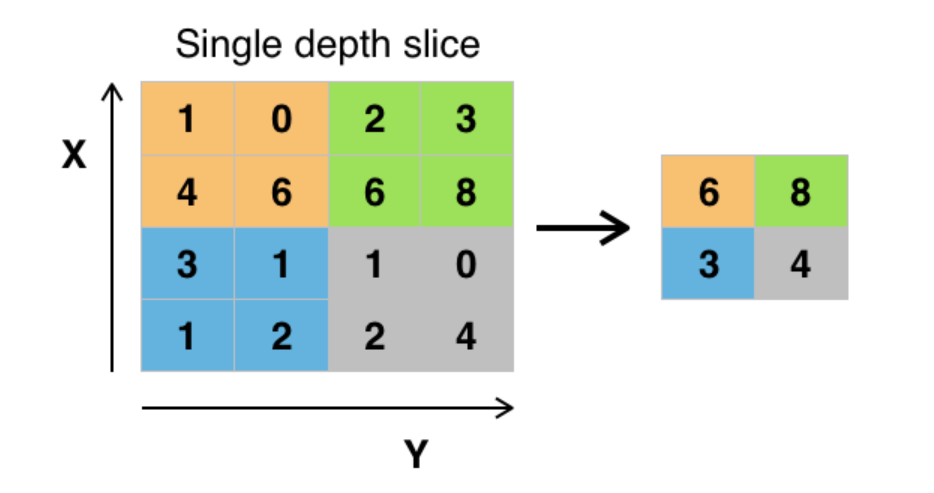#2019 iT 邦幫忙鐵人賽DAY 30
0
AI & Data

# 說明# 實作

TensorFlow提供tf.nn.max_pool（）函數以將最大池應用於卷積層。

``````...
conv_layer = tf.nn.conv2d(input, weight, strides=[1, 2, 2, 1], padding='SAME')
conv_layer = tf.nn.relu(conv_layer)
# Apply Max Pooling
conv_layer = tf.nn.max_pool(
conv_layer,
ksize=[1, 2, 2, 1],
strides=[1, 2, 2, 1],